Courses

# Test: Structural Analysis- 1

## 10 Questions MCQ Test GATE Civil Engineering (CE) 2022 Mock Test Series | Test: Structural Analysis- 1

Description
This mock test of Test: Structural Analysis- 1 for Civil Engineering (CE) helps you for every Civil Engineering (CE) entrance exam. This contains 10 Multiple Choice Questions for Civil Engineering (CE) Test: Structural Analysis- 1 (mcq) to study with solutions a complete question bank. The solved questions answers in this Test: Structural Analysis- 1 quiz give you a good mix of easy questions and tough questions. Civil Engineering (CE) students definitely take this Test: Structural Analysis- 1 exercise for a better result in the exam. You can find other Test: Structural Analysis- 1 extra questions, long questions & short questions for Civil Engineering (CE) on EduRev as well by searching above.
QUESTION: 1

### Match List - I (Method) with List-II (Factors) and select the correct answer using the codes given below the lists: List - I A. Moment distribution B. Slope deflection C. Kani’s method D. Force method List - II 1. Rotation factor 2. Flexibility 3. Hardy Cross 4. Displacements 5. Stiffness matrix

Solution:

Moment Distribution Method was developed by Hardy Cross
Slope Deflection is also known as Displacement Method.
Knai’s method involves use of rotation factor.
Force Method [Force] [flexibility matrix] = Deflection  Therefore, it involves use of flexibility matrix.

QUESTION: 2

### In the virtual work method of plastic analysis of steel structure, the virtual quantity is

Solution:

In the virtual work method of plastic analysis of steel structure, the virtual quantity is displacement.

Virtual work arises in the application of the principle of least action to the study of forces and movement of a mechanical system. The work of a force acting on a particle as it moves along a displacement will be different for different displacements. Among all the possible displacements that a particle may follow, called virtual displacements, one will minimize the action. This displacement is therefore the displacement followed by the particle according to the principle of least action. The work of a force on a particle along a virtual displacement is known as the virtual work.

Principle of virtual work: (unit-load Method)

Developed by Bernoulli: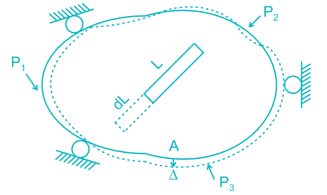To find Δ at point A du to loads P1, P2, P3. Remove all loads, apply virtual load P’ on point A.

For simplicity P’ = 1

It creates internal load u on representative element.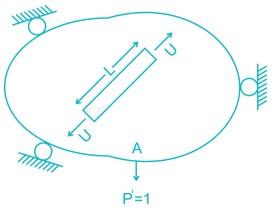Now remove this load, apply P1, P2, P3 due to which pt. A will be displaced by Δ

∴ External virtual work = 1.Δ

Internal virtual work = u.dL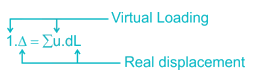P' = 1 = external virtual unit load in direction of Δ.

u = internal virtual load acting on element in direction of a dL.

Δ = external displacement caused by real loads.

dL = internal deformation caused by real loads.

QUESTION: 3

### The figure below shows the displacement caused by load P at two points 1 and 2 respectively. According to Maxwell reciprocal theorem which option is CORRECT.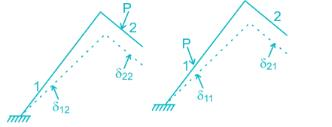Solution:

The Maxwell reciprocal theorem, states that the deflection at a point A in the direction of  1 due to load at point B in the direction of 2 is equal in the magnitude to the deflection of point B in the direction of 2 produced by a load applied at A in direction 1.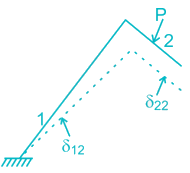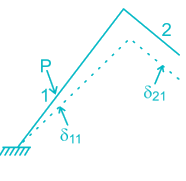Hence,

δ12 = δ21

QUESTION: 4

A fixed beam AB is subjected to a triangular load varying from zero at end A to ‘w’ per unit length at end B. The ratio of fixed end moment at B to A will be

Solution: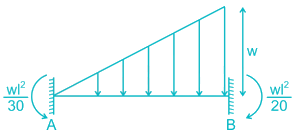The fixed end moment at end A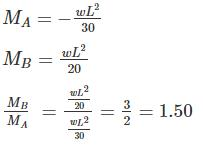QUESTION: 5

Find the carry over moment at support B, in the beam shown with internal hinges at C & D:-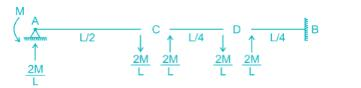Solution:

The beam has internal hinges at C & D, so the beam can be break down as shown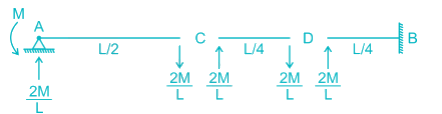In AC section moment applied = M

So the reaction will be = M/L/2 = 2/ML

The carry – over moment at B = 2M/L × L/4 = M/2

QUESTION: 6

The correct order of strain energy in the beams:-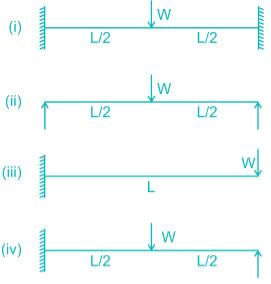Solution:

Deflection, δ = ∂U/∂F

In which beam deflection is higher, strain energy stored will be maximum.

In cantilever, the deflection will be highest.

The deflection of a simply supported beam is higher than propped cantilever as one of the support is fixed.

In a fixed beam, the deflection will be minimum.

So,

δcantilever >  δsimple-support > δpropped cantilever > δfixed

∴ Ufixed < Uproped-cantilever < Usimple-support < Ucantliver

QUESTION: 7

The strain energy stored (U) in the cantilever beam shown is –

Solution: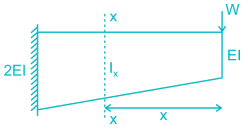BM at section X-X, Mx = W.x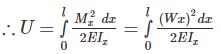Now, for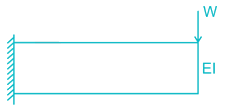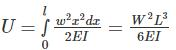For,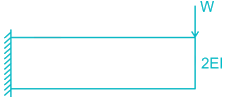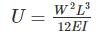∴ For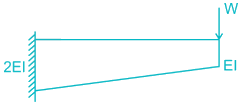U will be less than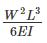but greater than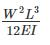QUESTION: 8

Find the vertical displacement of joint B if the spring constant k = 1 KN/mm and the rigid beam is loaded as shown below:-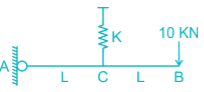Solution:

The FBD of the beam will be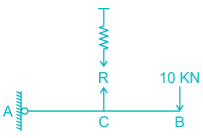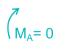R × L = 10 × 2L

R = 20 KN

So the extension of the spring will be

R = Kx

20 = 1. x

x = 20 mm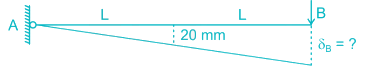From similar triangle

δB = 20 × 2 = 40 mm

QUESTION: 9

Find the rotation of joint B if the frame is loaded as shown below: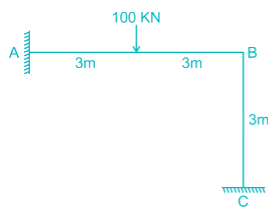Solution: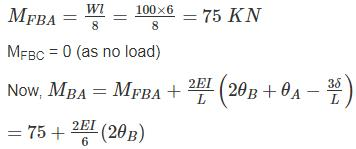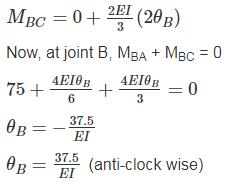QUESTION: 10

All members of the frame shown below have equal flexural rigidity EI. Calculate the rotation of joint O if moment M is applied?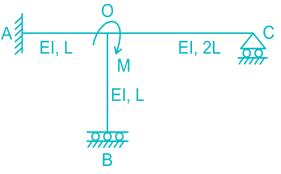Solution:

Concept :-

Stiffness value, K when far end is fixed = 4EI/L

Stiffness value, K when far end is roller = 3EI/L

Stiffness value, K when far end is guided roller = EI/L

Calculation:-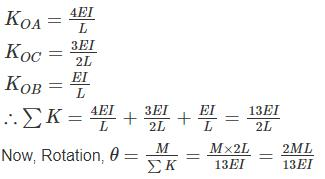For stiffness coefficient when far end is guided roller support refer
:-

Advanced Structural Analysis, Prof. Devdas MenonDepartment of Civil Engineering, Indian Institute of Technology, Madras

Module - 5.3 ,Lecture - 29Matrix Analysis of Beams and GridsPage No. - 9